MATLAB Function Referencebesselh

Bessel function of the third kind (Hankel function)

Syntax

• ```H = besselh(nu,K,Z)
H = besselh(nu,Z)
H = besselh(nu,K,Z,1)
[H,ierr] = besselh(...)
```

Definitions

The differential equation

•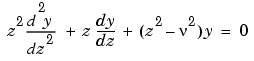where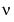is a nonnegative constant, is called Bessel's equation, and its solutions are known as Bessel functions.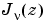and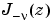form a fundamental set of solutions of Bessel's equation for noninteger.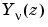is a second solution of Bessel's equation - linearly independent of- defined by

•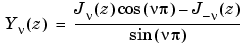The relationship between the Hankel and Bessel functions is

•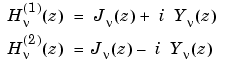whereis `besselj`, andis `bessely`.

Description

```H = besselh(nu,K,Z) ``` computes the Hankel function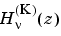, where `K` = 1 or 2, for each element of the complex array `Z`. If `nu` and `Z` are arrays of the same size, the result is also that size. If either input is a scalar, `besselh` expands it to the other input's size. If one input is a row vector and the other is a column vector, the result is a two-dimensional table of function values.

```H = besselh(nu,Z) ``` uses `K` = 1.

```H = besselh(nu,K,Z,1) ``` scalesby `exp(-i*Z)` if `K` = 1, and by `exp(+i*Z)` if `K` = 2.

```[H,ierr] = besselh(...) ``` also returns completion flags in an array the same size as `H`.

 ierr Description `0` `besselh` successfully computed the Hankel function for this element. `1` Illegal arguments. `2` Overflow. Returns `Inf`. `3` Some loss of accuracy in argument reduction. `4` Unacceptable loss of accuracy, `Z` or `nu` too large. `5` No convergence. Returns `NaN`.

Examples

This example generates the contour plots of the modulus and phase of the Hankel function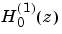shown on page 359 of  Abramowitz and Stegun, Handbook of Mathematical Functions.

It first generates the modulus contour plot

• ```[X,Y] = meshgrid(-4:0.025:2,-1.5:0.025:1.5);
H = besselh(0,1,X+i*Y);
contour(X,Y,abs(H),0:0.2:3.2), hold on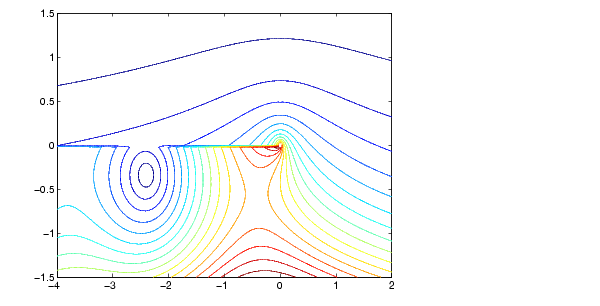```

then adds the contour plot of the phase of the same function.

• ```contour(X,Y,(180/pi)*angle(H),-180:10:180); hold off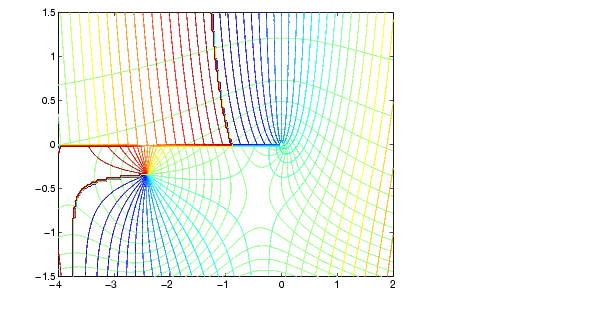```

See Also

`besselj`, `bessely`, `besseli`, `besselk`

References

  Abramowitz, M. and I. A. Stegun, Handbook of Mathematical Functions, National Bureau of Standards, Applied Math. Series #55, Dover Publications, 1965.

© 1994-2005 The MathWorks, Inc.